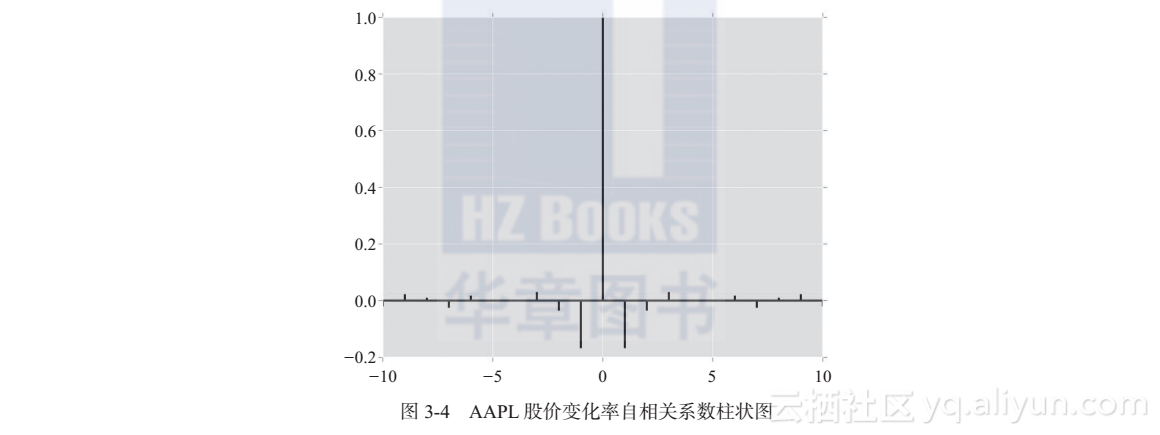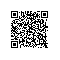# 《构建实时机器学习系统》一3.3　利用 Pandas 分析实时股票报价数据

### 3.3　利用 Pandas 分析实时股票报价数据

import pandas as pd
from datetime import datetime

#### 3.3.1　外部数据导入

data = pd.read_csv("aapl.csv",
names = ["timestamp_raw","Open","High",
"Low","Close","Volume"],
index_col = False)
print(type(data))

data.head(5)
data.tail(5)

UNIX_EPOCH = datetime(1970, 1, 1, 0, 0)
def ConvertTime(timestamp_raw, date):
""" 该函数会将原始的时间转化为所需的datetime格式 """
delta = datetime.utcfromtimestamp(timestamp_raw) - UNIX_EPOCH
return date + delta

data.index = map(lambda x: ConvertTime(x, datetime(2015, 8, 3)),
data["timestamp_raw"]/1000)

data = data.drop("timestamp_raw",1)

#### 3.3.2　数据分析基本操作

dir(data)

data.mean()

data.describe()

data.index.min()
data.index.max()

data_trading_hour = data["201508030930":"201508031529"]

#### 3.3.3　可视化操作

import matplotlib
from matplotlib import pyplot as plt

Matplotlib 自带的画图风格比较僵硬，需要改改，同时为了向 R 致敬，这里设置画图风格为 R ggplot 风，设置命令如下：

matplotlib.style.use('ggplot')

data_trading_hour["Close"].plot()
plt.show()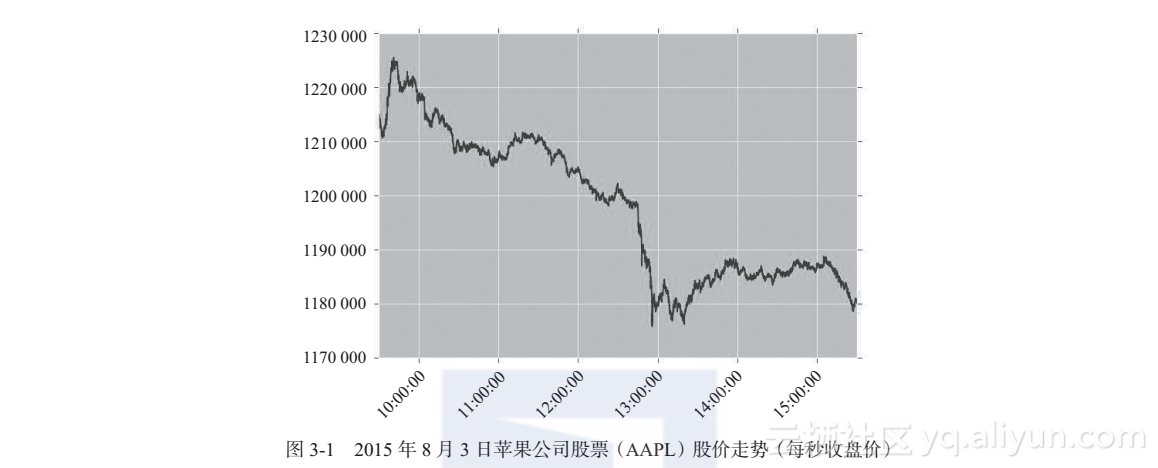data_trading_hour["Volume"].plot.hist()
plt.show()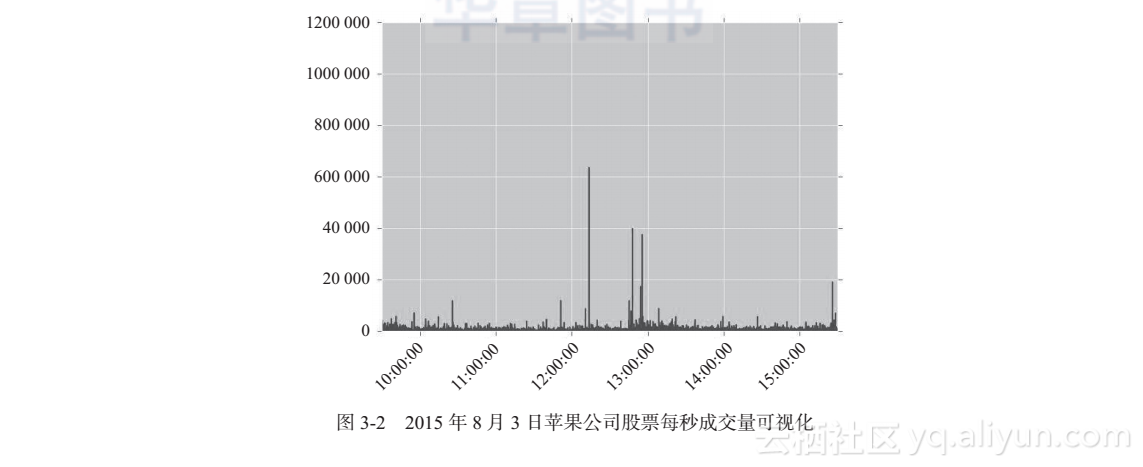data_trading_hour["Volume"].describe()
plt.show()

#### 3.3.4　秒级收盘价变化率初探

data_trading_hour["Close"].diff().plot.hist()
plt.show()

change.plot()
plt.show()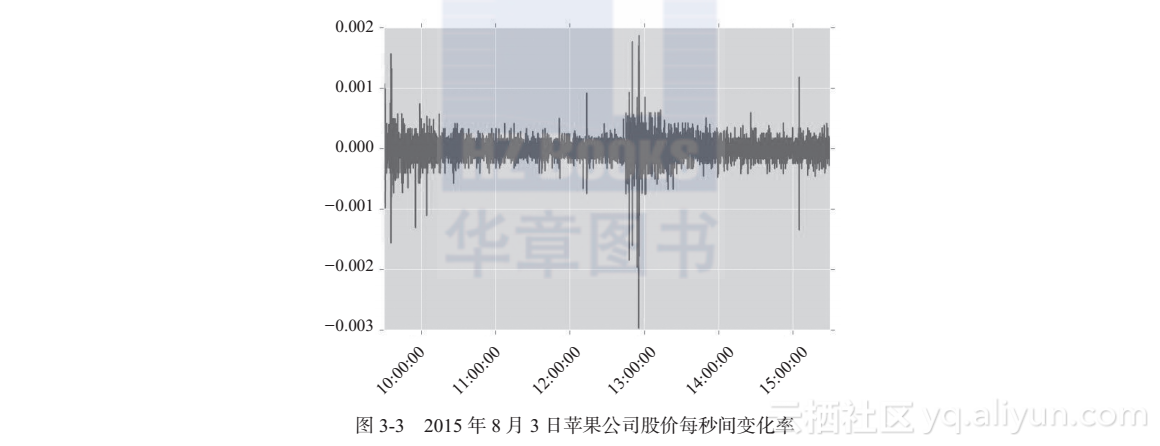change.shift(1).corr(change)
change.shift(2).corr(change)

plt.acorr(change[1:], lw = 2)
plt.show()